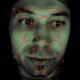# Sierpiński Glitch Texture #1

Actionscript:
1. [SWF(frameRate=60, backgroundColor=0x000000, width=500, height=500)]
2. var canvas:BitmapData = new BitmapData(500,500,false, 0x000000);
4. var clone:BitmapData = new BitmapData(500,500,false, 0x000000);
5. var canvasRect:Rectangle = canvas.rect;
6. var w:int = canvas.width;
7. var w2:Number = 1/w;
8. var w10:Number = 1/(w * 80);
9. var convert:Number = Math.PI/180;
10. var size:int = canvas.width * canvas.height;
11. var pix:Vector.<uint> = new Vector.<uint>(size, true);
12. var gray:ColorMatrixFilter = new ColorMatrixFilter([1, 0.55, 0.55, 0,0,0.55, 0.9, 0.55, 0,0,0.55, 0.55, 0.550,0, 0,0,0,1,0]);
13. var m:Matrix = new Matrix();
14. m.scale(1,-1);
15. m.translate(0,canvas.height);
16. var sin:Number = 0, cos:Number = 0;
17. var dx:Number = 0, dy:Number = 0;
18. var pnt:Point = new Point();
19. var blur:BlurFilter = new BlurFilter(10,10,1);
21. function onLoop(evt:Event):void {
22.     canvas.lock();
23.     dx += (mouseX * 10 - 3000 - dx) / 8;
24.     dy += (mouseY * 4 - dy) / 8;
25.     for (var i:int = 0; i<size; i++){
26.         var xp:int = i % w;
27.         var yp:int = int(i * w2);
28.         var xp2:int = xp <<1;
29.         var t:Number;
30.         t = ((yp|xp) * (xp + dx) *w10) % 6.14687;
31.
32.         //compute sine
33.         // technique from http://lab.polygonal.de/2007/07/18/fast-and-accurate-sinecosine-approximation/
34.         // by Michael Baczynski
35.         if (t<0) {
36.             sin=1.27323954*t+.405284735*t*t;
37.         } else {
38.             sin=1.27323954*t-0.405284735*t*t;
39.         }
40.         // compute cosine
41.         t = (xp2 + dy) * convert % 6.28;
42.         t+=1.57079632;
43.         if (t>3.14159265) {
44.             t-=6.28318531;
45.         }
46.         if (t<0) {
47.             cos=1.27323954*t+0.405284735*t*t;
48.         } else {
49.             cos=1.27323954*t-0.405284735*t*t;
50.         }
51.         var c1:int = 31 * (sin - cos);
52.         if (c1 <0) c1 = 256 - c1;
53.         c1 = (c1 <<3 | c1) ;
54.         pix[i] = c1 <<15 | c1 <<8 | c1;
55.     }
56.     canvas.setVector(canvasRect, pix);
57.     clone.copyPixels(canvas, canvasRect, pnt);
58.     canvas.draw(clone, m, null, BlendMode.SUBTRACT);
59.     clone.copyPixels(canvas, canvasRect, pnt);
60.     clone.applyFilter(clone, canvasRect, pnt, blur);
62.     canvas.unlock();
63. }

More strange real-time texture generation. This one is particularly glitchy looking with hints of Sierpiński.

Check out the swf at wonderfl.net...1.DieTapete
Posted August 19, 2009 at 11:04 pm | Permalink

Look’s really cool! I think this is the first time that I like something sierpinskiesque.

2.Valentin
Posted August 20, 2009 at 12:42 am | Permalink

hm
this is cool
don’t you have a broken tv distortion effect I could use? q:

3.Og2t
Posted August 20, 2009 at 5:14 am | Permalink

yeah, that’s very TV like – I like it! Respect for using ń in Wacław Sierpiński’s surname4.Zevan
Posted August 20, 2009 at 7:30 am | Permalink

@DieTapete thanks.
@Valentin I don’t have one, but you could use some of the info on this site to create your own, using setPixel/setVector and a displacement map filter.

@Og2t - heh, yeah gotta use the ń.